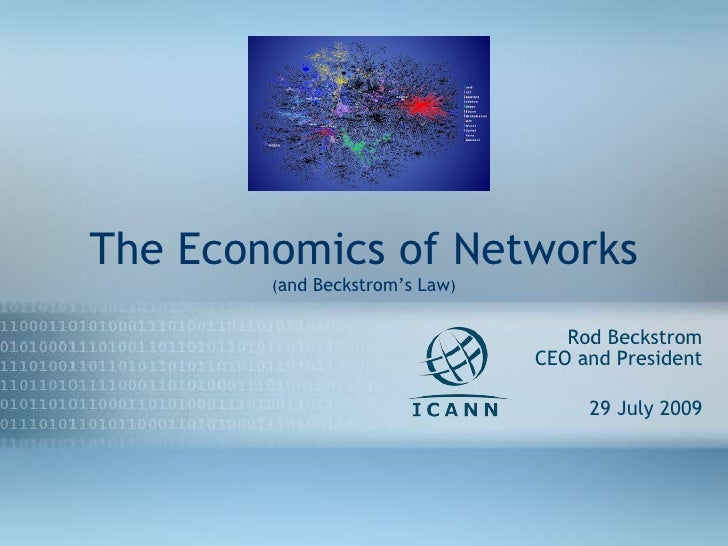Successfully reported this slideshow.Upcoming SlideShare
×

# Beckstrom's Law & The Economics Of Networks - ICANN

16,564 views

Published on

Beckstrom's Law - The Economics of Networks presented at BlackHat Las Vegas, July 29, 2009. Presents a universal transaction based model for valuing any network- technical or social.

Also presentes "The Network Effect" mathematically as well as introducing "The Inverse Network Effect."

Also presents Metcalfe's Law and uses fax network to demonstrate that it doesn't work.

To see the related presentation on video, go to YouTube or to the www.blackhat.com websites.

Published in: Technology, Economy & Finance
• Full Name
Comment goes here.

Are you sure you want to Yes No• Smart presentation.

Are you sure you want to  Yes  No

### Beckstrom's Law & The Economics Of Networks - ICANN

1. The Economics of Networks ( and Beckstrom’s Law ) Rod Beckstrom CEO and President 29 July 2009 27Apr08
3. 29Jul09 Participating in the Internet eco-system and supporting it
4. Do network economics matter? Value of network to one user Total value of a network Security economics Security risk management Hacker economics Economics of deterrence Supply chain incentives Economics of Internet protocols (architecture) Economics of outages Economics of resiliency (correlation of losses) 29Jul09
5. Metcalfe’s Law 29Jul09 fax networks is larger today than ever, but less valuable because of substitutes such as email, Fedex, UPS, PDF’s, web, etc., thus the model does not hold Where: V = value of network j n = the number of end points or nodes p = some constant V j = n 2 * p Historic Network Value Models
6. t not n ! 29Jul09
7. t not n ! 29Jul09
8. New Model: Beckstrom’s Law Economics of Networks 29Jul09 The value of a network equals the net value added to each user’s transactions, summed for all users.
9. V i,j = ΣB - ΣC Where: V = value of network j to user i B = the benefit value of all transactions C = the cost of all transactions Value of a Network to One User New Network Model 29Jul09
10. V = B - C V = \$26 - \$16 V = \$10 If it costs \$26 to buy the book in a store or \$16 to buy it over the Internet, including shipping, the net value is \$10. Online Book Purchase Case 29Jul09
11. V[j] = ΣV i,j = ΣB - ΣC Network Value is Summation of Value to All Users New Network Model 29Jul09
12. 29J ul09 Where: ΣV i,j = value of a network j to all users V i,j = net present value of all transactions to user i with respect to network j, over any time period j = identifies one network i = one user of the network B i,k = the benefit value of transaction k to individual i C i,l = the cost of transaction l to individual i r k and r l = the discount rate of interest to the time of transaction k or l t k or t l = the elapsed time in years to transaction k or l n,m,p = maximum number of individuals i and transactions k and l Beckstrom’s Law New Network Model
13. The “Network Effect” 29Jul09
14. For those networks where… The “Network Effect” 29Jul09 More users add to more total value
15. For those networks where… The “Inverse Network Effect” 29Jul09 More users reduce total value <